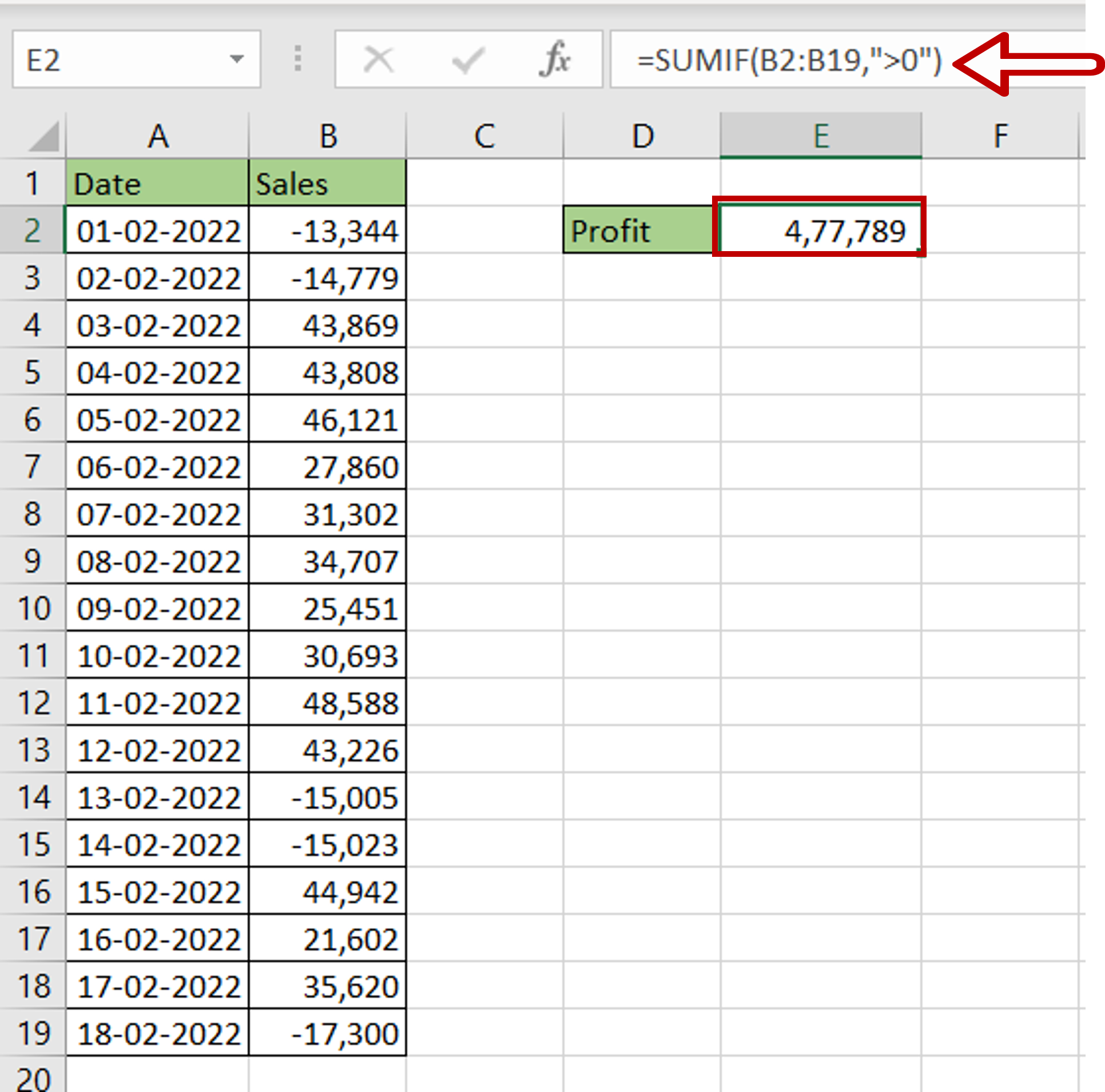# How to sum only positive numbers in Excel

You can watch a video tutorial here.

One of the great things about Excel is the ease with which complex and simple mathematical operations can be done. Excel has several tools and functions that can be used to aggregate numbers. There may be a situation where you want to add up only the positive numbers in a list of numbers. For example, you have the sales of an outlet that has shown both positive and negative numbers. You would like to sum only the positive numbers.In Excel, this can be done using the SUMIF() function.

1. SUMIF() function: this adds numbers based on a criteria
a. Syntax: SUMIF(criteria range, criteria, sum_range)
i. criteria range: the range to be evaluated by the criteria
ii. criteria: the criteria
iii. sum_range: the range to be added. This is optional and needs to be given only if it is different from the criteria range

### Step 1 – Create the formula– In the destination cell, enter the formula using cell references:
= SUMIF(Sales,”>0”)
– Press Enter